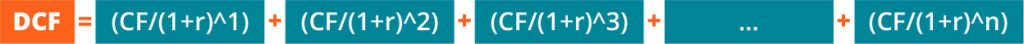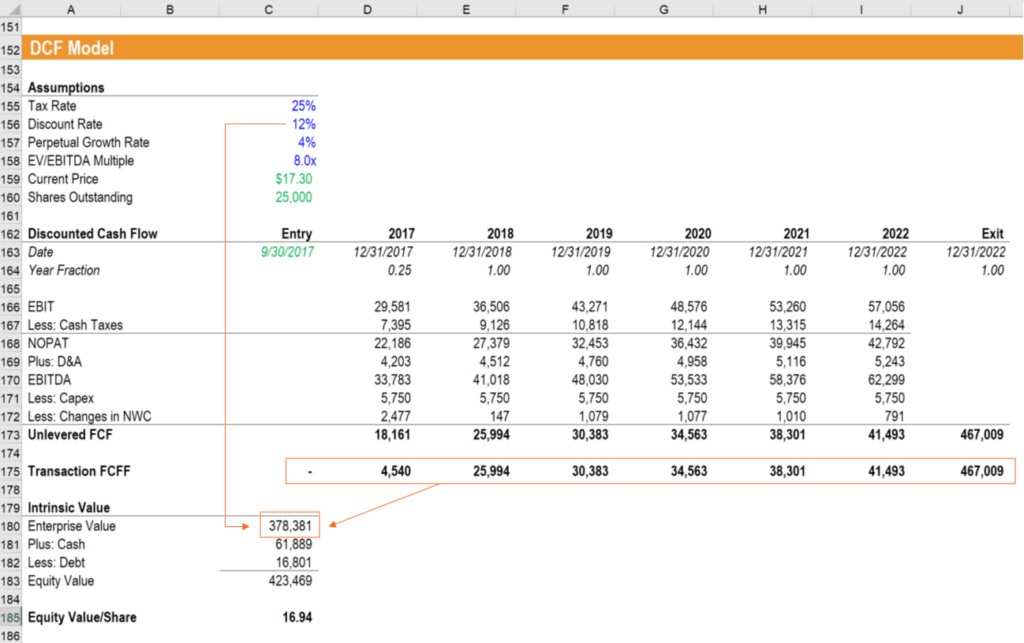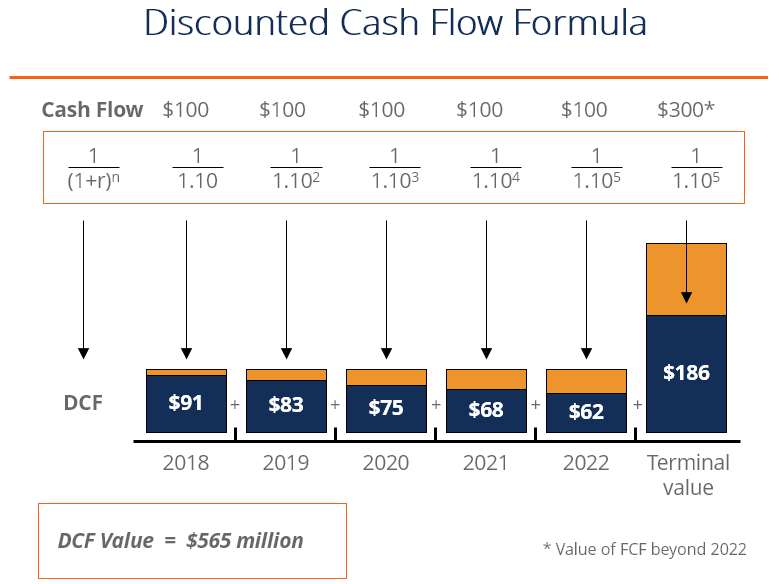# Discounted Cash Flow DCF Formula

How to calculate net present value

## Guide to the Discounted Cash Flow DCF Formula

This article breaks down the discounted cash flow DCF formula into simple terms.  We will take you through the calculation step by step so you can easily calculate it on your own. The DCF formula is required in financial modeling to determine the value of a business when building a DCF Model in Excel.

Watch this short video explanation of how the DCF formula works.

Video: CFI’s free Intro to Corporate Finance Course.

### What is the Discounted Cash Flow DCF Formula?

The discounted cash flow (DCF) formula is equal to the sum of the cash flow in each period divided by one plus the discount rate (WACC) raised to the power of the period number.

Here is the DCF formula:Where:

CF = Cash Flow in the Period

r = the interest rate or discount rate

n = the period number

### Analyzing the Components of the Formula

##### 1. Cash Flow (CF)Cash Flow (CF) represents the free cash payments an investor receives in a given period for owning a given security (bonds, shares, etc.)

When building a financial model of a company, the CF is typically what’s known as unlevered free cash flow.  When valuing a bond, the CF would be interest and or principal payments.

##### 2. Discount Rate (r)For business valuation purposes, the discount rate is typically a firm’s Weighted Average Cost of Capital (WACC).  Investors use WACC because it represents the required rate of return that investors expect from investing in the company.

For a bond, the discount rate would be equal to the interest rate on the security.

##### 3. Period Number (n)Each cash flow is associated with a time period. Common time periods are years, quarters, or months.  The time periods may be equal, or they may be different.  If they’re different, they’re expressed as a decimal.

### What is the DCF Formula Used For?

The DCF formula is used to determine the value of a business or a security.   It represents the value an investor would be willing to pay for an investment, given a required rate of return on their investment (the discount rate).

#### Examples of Uses for the DCF Formula:

• To value an entire business
• To value a project or investment within a company
• To value a bond
• To value shares in a company
• To value an income-producing property
• To value the benefit of a cost-saving initiative at a company
• To value anything that produces (or has an impact on) cash flow

Below is a screenshot of the DCF formula being used in a financial model to value a business.  The Enterprise Value of the business is calculated using the =NPV() function along with the discount rate of 12% and the Free Cash Flow to the Firm (FCFF) in each of the forecast periods, plus the terminal value.Image: CFI’s Business Valuation Modeling Course.

### What Does the Discounted Cash Flow Formula Tell You?

When assessing a potential investment, it’s important to take into account the time value of money, or the required rate of return that you expect to receive.

The DCF formula takes into account how much return you expect to earn, and the resulting value is how much you would be willing to pay for something, to receive exactly that rate of return.

If you pay less than the DCF value, your rate of return will be higher than the discount rate.

If you pay more than the DCF value, your rate of return will be lower than the discount.

### Illustration of the DCF Formula

Below is an illustration of how the discounted cash flow DCF formula works.  As you will see in the example below, the value of equal cash flow payments is being reduced over time, as the effect of discounting impacts the cash flows.Image: CFI’s free Intro to Corporate Finance Course.

### Terminal Value

When valuing a business, the forecasted cash flow typically extends about 5 years into the future, at which point a terminal value is used.  The reason is that it becomes hard to make a reliable estimate of how a business will perform that far in the future.

There are two common methods of calculating the terminal value:

• Exit multiple (where the business is assumed to be sold)
• Perpetual growth (where the business is assumed to grow at a reasonable, fixed growth rate forever)

Check out our guide on how to calculate the DCF terminal value to learn more.

### DCF vs NPV

The total Discounted Cash Flow (DCF) of an investment is also referred to as the Net Present Value (NPV).  If we break the term NPV we can see why this is the case:

Net = the sum of all positive and negative cash flows

Present value = discounted back to the time of the investment

### DCF Formula in Excel

MS Excel has two formulas that can be used to calculate discounted cash flow, which it terms as “NPV”.

Regular NPV formula:

=NPV(discount rate, series of cash flows)

This formula assumes that all cash flows received are spread over equal time periods, whether years, quarters, months, or otherwise.  The discount rate has to correspond to the cash flow periods, so an annual discount rate of 10% would apply to annual cash flows.

=XNPV(discount rate, series of all cash flows, dates of all cash flows)

With XNPV it’s possible to discount cash flows that are received over irregular time periods.  This is particularly useful in financial modeling when a company may be acquired partway through a year.

For example, this initial investment may be on August 15th, the next cash flow on December 31st, and every other cash flow thereafter a year apart. XNPV can allow you to easily solve for this in Excel.

To learn more, see our guide on XNPV vs NPV in Excel.

CFI’s mission is to help you advance your career.  With that mission in mind, we’ve compiled a wide range of helpful resources to guide you along your path to becoming a Certified Financial Modeling Analyst.

Relevant resources include:

• Internal Rate of Return
• Valuation Methods
• DCF Modeling Tips
• Financial Modeling Best Practices

### Valuation Techniques

Learn the most important valuation techniques in CFI’s Business Valuation course!

Step by step instruction on how the professionals on Wall Street value a company.

Learn valuation the easy way with templates and step by step instruction!